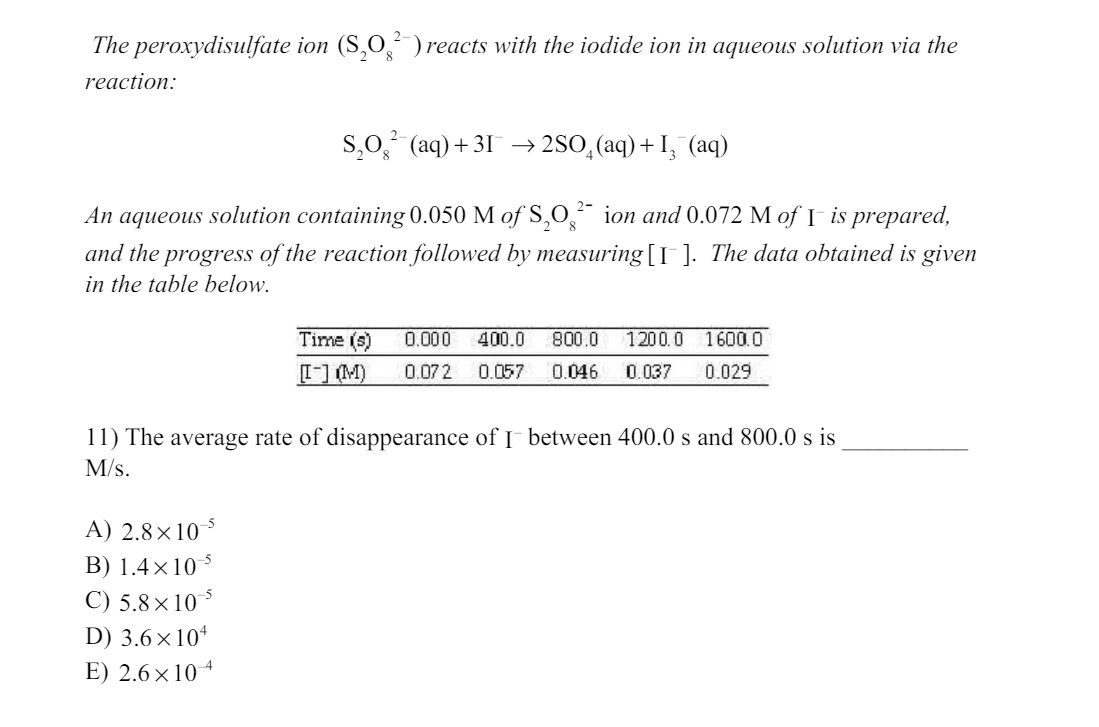# ratesI am really confused about this problem. In one of the lessons, it said to find the average rate I should set up the equation as -1d[I^-]/3d[T]. with the 3 on the bottom because the coefficient of I^-1 is 3. rate = -∆C/3∆t = (0.045 M - 0.057 M)/3(800 s -400 s)=-.9.2e-6. However, this is not one of the choices. Can you explain why it is A?